Module 8 - Continuity Introduction | Lesson 1 | Lesson 2 | Lesson 3 | Self-Test Self Test Which of the following functions are continuous at the given point?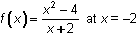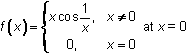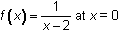What type of discontinuity does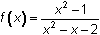have at x = -1? Illustrate the discontinuity with a graph.   Let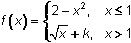. For what value of k will f(x) be continuous at x = 1?   Graph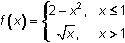using Boolean expressions.   Redefine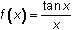so that it is continuous at x = 0.   Click here to check your answers. < Back ©Copyright 2007 All rights reserved. | Trademarks | Privacy Policy | Link Policy